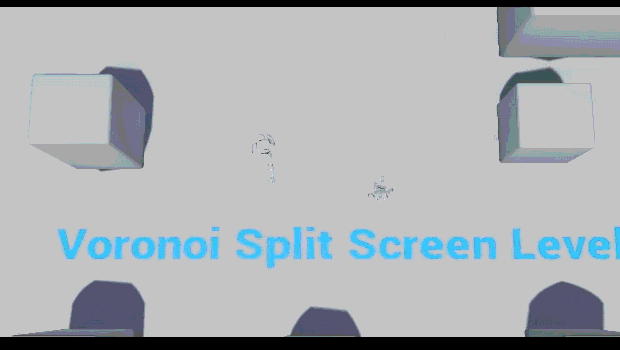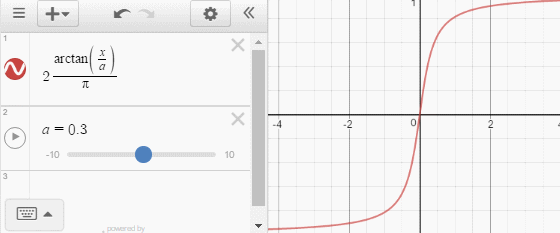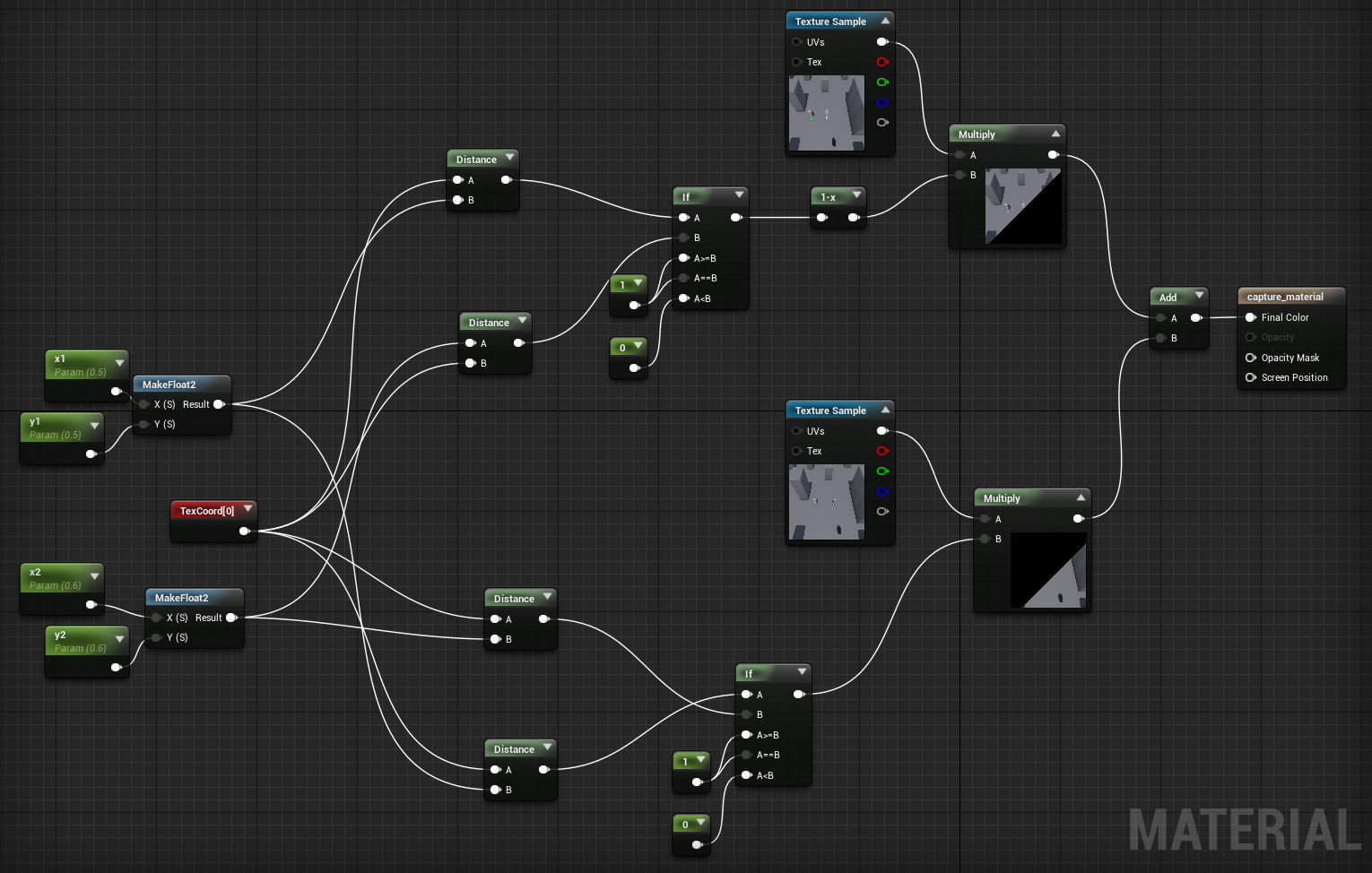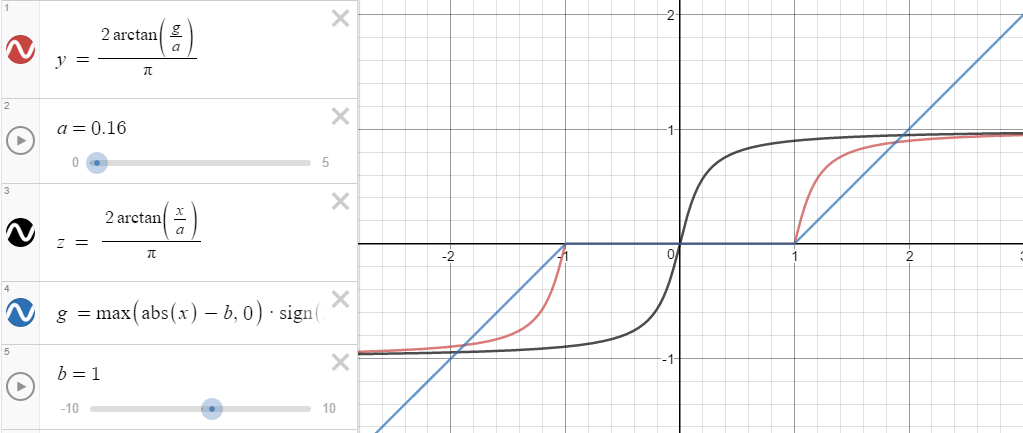### What A Voronoi Split Screen System IsA voronoi diagram is one where nodes (characters in this screenshot) are separated into spaces that are divided by a line, where every point on the line is an equal distance from both nodes.

For this tutorial, we are only looking at diagrams of two nodes and therefore one line.

This voronoi split screen system moves the line so that it is always between the two players, which gives them a constant understanding of where they are in relation to each other, and it merges the screen into one when they are within a certain distance of each other.

### Player Location → Voronoi Nodes

First, we want to translate the players' positions to the node positions.Where `x` is the distance from the midpoint `M` between the two characters, to one of the players. And `y` is the distance from the center of the screen to the node.

We will keep the angle the same, and so we'll only deal with the distance, `y`. We need to keep the node on the screen, so we need to translate a distance (which could go to infinity) to a finite range, and we want to get there gradually. The following function should do the job:We can dial `a` up and down to change the curve amount, and this will get us to the perfect range, 0 to 1. That range is perfect because we can simply multiply `y` by a constant, and now our function goes from 0 to that constant. So we can dial `a` to be the max distance we want our nodes to be from the center of the screen, and our nodes will gradually get further apart as the players move apart, but well never go off the screen.

With this, we have translated the two players' positions from player-space to screen-space.

### Splitting The Screen

Now we need to actually split the screen.

Without going into too much detail, I made an unreal shader that, for each pixel, checks to see which player it is closer to, and renders that player's view. This is a simplified version of it where [x1, y1], [x2, y2] are the coordinates of the nodes in screen-space.This is then rendered onto the screen, which gives this lovely result:

Two player voronoi split screen is working!@UnrealEngine #UE4 #indiedev #gamedev pic.twitter.com/LuN0ThUVVJ

— Matt Woelk (@MattWoelk) July 12, 2016

### Dividing Line Thickness and Damping

This is wonderful! But we still need to separate the screens with a line, as it's a bit tough to tell what's going on. Let's add a line in between, and let's vary the thickness based on the distance between the nodes.

To draw a black line, we simply calculate which view to draw based on the distance from the present node to a point that is one line-thickness closer than the other node. The space not drawn by either view is drawn as black, which becomes the line:

Variable-width dividing line for the voronoi split-screen.@UnrealEngine #UE4 #gamedev

— Matt Woelk (@MattWoelk) July 21, 2016

Instead of putting the distance between nodes straight into the shader, we can modify how quickly the line expands by putting that distance through this function:

— Matt Woelk (@MattWoelk) September 8, 2016

With this damping function, the line will grow more gradually, which will give information about the distance of the characters at even further distances rather than expanding so quickly. (As I write this, I realize this function could probably have stood in for the arctan one above, which could simplify things a bit.)

The last step is to get the views to merge together.

Instead of putting the distance of the players straight into the shader, we can put it through a function that has a buffer zone, so it stays at 0 for a given time, and then scales as normal. You're probably seeing the pattern by now: find the simplest function that will do what you need, and then pass it through other functions ("compose" functions) to change its behaviour. Here's how this dead zone function looks:The black line is the original function, the blue line is the dead zone function, and the red line is the result. `b` changes the size of the dead zone.

If you run this comparison componentwise (`x` by itself, and then `y` by itself) then you get a line that sticks at horizontal and vertical when it's close:

Let's go explore!#screenshotsaturday #UE4 #gamedev pic.twitter.com/8r6txxJU1D

— Matt Woelk (@MattWoelk) September 3, 2016

And if you compare using vectors you get a smooth transition, like we had before implementing a dead zone: